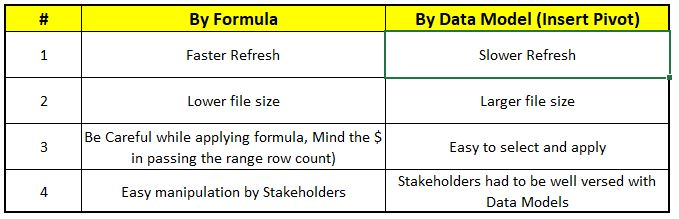Data Analytics

# How to Count Distinct in a pivot table?

Hello Learners,

Today, I am going to talk about a handy and simple Excel formula which can help you count distinct values in a pivot table. When we create a pivot table and look for field values options we can see that we can summarize values by Count, Sum, average but there is no option for count distinct.To overcome this limitation, an easy trick is to create a calculated column in the source range by using the below formula.Copy Formula Here>>        =IF(COUNTIF(\$B\$3:\$B3,B3)=1,1,0)